# Formulas and Structured Data in Excel Tables

I created a data table on a worksheet, then converted it to an Excel Table. Before the conversion, I had entered a formula for Gross Product in the last column. This formula looks like most formulas do in Excel, with cell references, as pictured below. The Gross Product formula in cell L5 is =K5+J5+I5.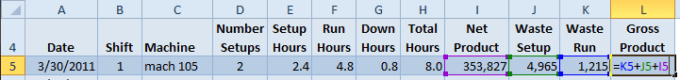The great thing about converting to a Table is that when I add more data to the table, the formula is automatically copied down for me.

If I change this formula, then Excel will automatically copy the change to all rows in the column. And it doesn’t matter if I change the first cell at the top of the column or change a cell in the middle, all rows in the column will be modified.

## Using Column References in a Table Formula

Tables have a structured data format you can see when adding a formula after the Table has been created. In my example I will reenter the Gross Product formula so that it equals Net Product plus Waste Setup plus Waste Run. Instead of cell references, Excel uses column references for the Table.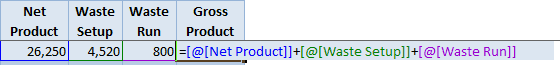The formula =[@[Net Product]]+[@[Waste Setup]]+[@[Waste Run]] was entered in row 18 of the Table and was immediately copied to all rows in the column when I pressed Enter.

Notice that each column reference in the formula is located between square brackets [ ]. The @ sign refers to “this row” and is also included in square brackets. So the formula in cell L5 for Gross Product equals the values for Net Product in row 5 plus Waste Setup in row 5 plus Waste Run in row 5.

An equivalent formula using the SUM function is =SUM(Table1[@[Net Product]:[Waste Run]]).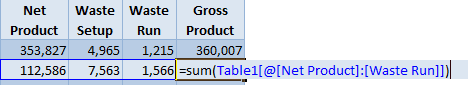Again, when I changed the formula in row 6, it was copied to all rows in the Gross Product column.

## Formulas Outside the Table Range

As you may recall, Table Names in Excel are like range names and show up in formula autocomplete lists. The structured column references also show up in formula autocomplete lists.

I want to calculate the average hours per setup in the Table so I use the formula =SUM(Table1[Setup Hours])/SUM(Table1[Number Setups]) in cell E2, which is outside the Table range. Formula autocomplete helps me build the formula by listing all the columns in the Table — after I type the Table name followed by an open square bracket [.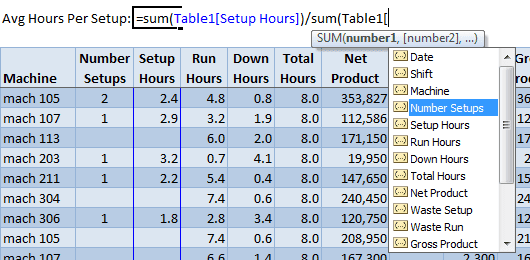Notice the Table1 name precedes each column reference. You have to remember the first few letters of the Table name so it will show up in the formula autocomplete list. Once the Table name is entered you have to remember to type an open square bracket [ to bring up the autocomplete list for the columns.

Or you can simply use the mouse to select the columns you want in the formula and the column syntax is entered automatically.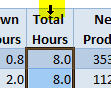Using a formula that references an entire Table column will expand to include any and all data for that column.

The same thing goes if I reference the Table in a formula. If I want to know the total number of rows in  the Table, I can simply enter =ROWS(Table1) into a cell and it will always give the correct number. (Filtering the Table has no effect on this formula.)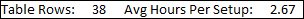Tables have a built in Total Row feature that we’ll cover in my next post on Tables.

### 2 thoughts on “Formulas and Structured Data in Excel Tables”

1.Dear Sir,
Pls. help me i have presence of 51 agents in excel -7 sheet
i want to count total presence on right hand coloumn of each one
e.g.
A;- P P P P A total present in month =?
B: A P P A P

•Assume you have the first set of characters in Cells A1:A5 which are P P P P A and you want to count the number of A’s. The formula would be =COUNTIF(A1:A5,”A”) which would return 1. If the second set of characters is entered into cells B1:B5 the formula =COUNTIF(B1:B5,”A”) would return 2.

I’m hoping this helps you.